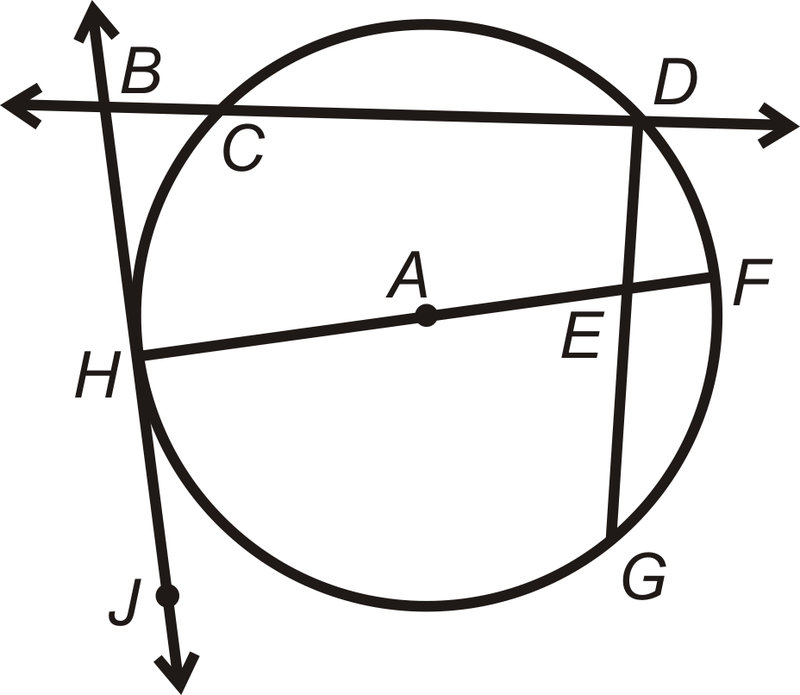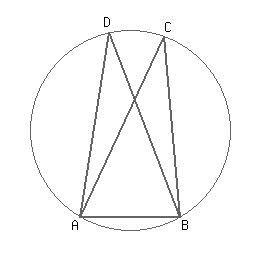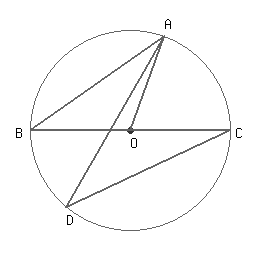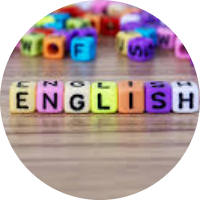# Circles and the Concepts of Geometry

This set of MCQs helps you brush up on important math topics and prepare you to dive into skill practice.

Start Quiz

In circle X, chords AB and CD are congruent and AB is 9 units from X. Find the distance from CD to X.

18 units

4.5 units

9 units

27 units

How many chords?1

2

3

0

The region between the chord and either of the arcs is called____.

A Segment

An arc

A sector

A semi

An angle whose vertex is the center of a circle,

Minor arc

Central angle

Major arc

Inscribed angle

If ∠DAB = 81° and ∠ABD = 69°, the value of ∠ACB is ___°.30

45

37

60

The bisectors of opposite angles of a cyclic quadrilateral ABCD intersect the circle circumscribing it at the points P and Q. If radius of the circle is 18 cm, find the distance between points P and Q.

40 cm

9 cm

18 cm

36 cm

Segments with endpoints on a circle are called _____.

Arcs

Polygons

Chords

If BC is the diameter of the circle and ∠BAO = 35°. Then find the value of ∠ADC.35°

45°

30°

60°

Quiz/Test Summary
Title: Circles and the Concepts of Geometry
Questions: 8
Contributed by: